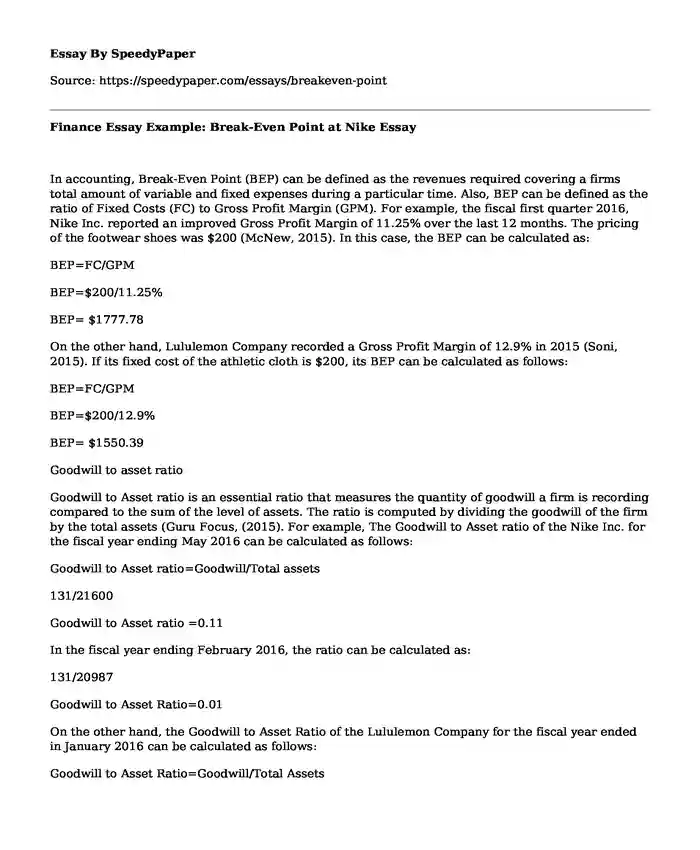# Finance Essay Example: Break-Even Point at Nike

Published: 2019-10-01 03:52:26Categories: Finance Nike Pages: 3 Wordcount: 619 words
143 views

In accounting, Break-Even Point (BEP) can be defined as the revenues required covering a firms total amount of variable and fixed expenses during a particular time. Also, BEP can be defined as the ratio of Fixed Costs (FC) to Gross Profit Margin (GPM). For example, the fiscal first quarter 2016, Nike Inc. reported an improved Gross Profit Margin of 11.25% over the last 12 months. The pricing of the footwear shoes was \$200 (McNew, 2015). In this case, the BEP can be calculated as:

Is your time best spent reading someone else’s essay? Get a 100% original essay FROM A CERTIFIED WRITER!

BEP=FC/GPM

BEP=\$200/11.25%

BEP= \$1777.78

On the other hand, Lululemon Company recorded a Gross Profit Margin of 12.9% in 2015 (Soni, 2015). If its fixed cost of the athletic cloth is \$200, its BEP can be calculated as follows:

BEP=FC/GPM

BEP=\$200/12.9%

BEP= \$1550.39

Goodwill to asset ratio

Goodwill to Asset ratio is an essential ratio that measures the quantity of goodwill a firm is recording compared to the sum of the level of assets. The ratio is computed by dividing the goodwill of the firm by the total assets (Guru Focus, (2015). For example, The Goodwill to Asset ratio of the Nike Inc. for the fiscal year ending May 2016 can be calculated as follows:

Goodwill to Asset ratio=Goodwill/Total assets

131/21600

Goodwill to Asset ratio =0.11

In the fiscal year ending February 2016, the ratio can be calculated as:

131/20987

Goodwill to Asset Ratio=0.01

On the other hand, the Goodwill to Asset Ratio of the Lululemon Company for the fiscal year ended in January 2016 can be calculated as follows:

Goodwill to Asset Ratio=Goodwill/Total Assets

23.83/1314.077

=0.02

The Goodwill to asset ratio for the quarter that ended in April 2016 can be calculated as follows:

24.49/1401.655

=0.02

Interest expense to debt ratio

Interest coverage can be defined as the ratio that determines how a firm can repay its interest expenses on the outstanding debts. For example, Nike Incs interest to debt ratio for the fiscal year ending Feb. 2016 can be calculated as follows:

Interest coverage=-1

Operating income=1123

-1*1123

=-1123

Interest expense=-5

Interest to debt ratio=-1123/-5

=224.60

On the other hand, the interest expense to debt ratio for the Lululemon Company indicated \$0. The fiscal year ended in April 2016 had an interest to debt ratio of \$0, \$0 for January 2016, and \$0 for April 2016. (\$0 + \$0 + \$0) = \$0. This shows that the company had no debt (Guru Focus, 2015).

Investment turnover measures how fast a firm turns over its current assets via its sales. For example, Nike Incs investment turnover for the fiscal year May 2015 can be calculated as follows:

=Sales/Average Total Assets

Revenue/{Total assets (May, 2014) + Total assets (May, 2015)/2}

(18594+21600)/2

30601/20097

=1.52

On the other hand, Investment turnover measures how fast a firm turns over its current assets via its sales. For example, Nike Incs investment turnover for the fiscal year May, 2015 can be calculated as follows:

=Sales/Average Total assets

Revenue/{Total assets (May, 2014) + Total assets (May, 2015)/2}

(1296.213+1314.077)/2

2060.523/1305.145

=1.58

This shows that investment turnover for Lululemon Inc. is higher compared to investment turnover of the Nike Inc. based on the fiscal years ending May 2014 and May 2015.

N/B: It should be noted that in this accounting analysis paper, all the depicted data are in millions except ratio and share data.

References

Guru Focus, (2015). Nike Inc. (NYSE:NKE) Goodwill. http://www.gurufocus.com/term/goodwill2asset/LULU/Goodwill%2Bto%2BAsset%2BRatio/Lululemon%2BAthletica%2BInc

Guru Focus, (2015). Nike Inc. (NYSE:NKE) Goodwill. http://www.gurufocus.com/term/Goodwill/NKE/nbsp%25253Bnbsp%25253B%252BGoodwill/Nike%2BInc

Guru Focus, (2015). Nike Inc. (NYSE:NKE) Interest Expense. http://www.gurufocus.com/term/InterestExpense/LULU/Interest%2BExpense/Lululemon%2BAthletica%2BInc

McNew, S. (2015). 4 Reasons Nike Inc. Has Such a High-Profit Margin. Profit Margins at the Leading Sports Apparel Retailer are some of the Best in the industry. How is this possible? http://www.fool.com/investing/general/2015/10/29/4-reasons-nike-inc-has-such-a-high-profit-margin.aspxSoni, P. (2015). Analyzing Lululemon Athleticas Market-Moving Fiscal 2015 Results. Market Realist. http://marketrealist.com/2015/04/analyzing-lululemon-athleticas-market-moving-fiscal-2015-results/

Finance Essay Example: Break-Even Point at Nike. (2019, Oct 01). Retrieved from https://speedypaper.com/essays/breakeven-point

Request Removal

If you are the original author of this essay and no longer wish to have it published on the SpeedyPaper website, please click below to request its removal:

Liked this essay sample but need an original one?

Hire a professional with VAST experience!